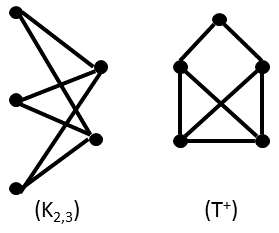## December 2015 solution

#### Part 1:

C3 is also known as K3, the clique of three vertices. C3 appears as the most common induced subgraph in K6, the clique of size 6 (i.e., 6 vertices with all 15 possible edges between them).

To see this, note that picking any n vertices will lead to a Kn induced subgraph. There are more ways to pick 3 of 6 than to pick any other number from 6.

#### Part 2:

There are no graphs that have C4 as their most common induced subgraph.

Suppose, to the contrary, that such a graph, G, had existed.

Let L(G) be the set of L2 induced subgraphs in G. (L2 is the path of length 2: three vertices connected by 2 edges.) Let S(x), for x in L(G), be the number of distinct ways x can be completed to a C4 by the addition of a vertex. Finally, let Xi be the number of x in L(G) for which S(x)=i.

Using the Xi we can count the number of C4 copies.

#(C4) = Σi Xi i/4

The reason we need to divide by four is because each square is counted four times (due to symmetries): each of its four induced copies of L2 can be expanded into a C4.

However, we can also count other subgraphs in the same way. The simplest of these is the count of L2 copies.

#(L2) = Σi Xi

Similarly, for each x in L(G) we can create a graph by picking two of its possible completions into a square, instead of just one. This means picking a shape with 5 vertices: the original 3 plus 2 new ones. The shape of the resulting graph can be one of two, depending on whether the two new vertices are connected by an edge or not.

We call the shape without an extra edge K2,3 and the one with an extra edge T+.Together, we have the equation

3#(K2,3)+#(T+) = Σi Xi i(i-1)/2

Here, i(i-1)/2 signifies the number of ways to pick pairs from i items, and the multiplication by 3 comes, again, from symmetries.

Consider now the following equation.

#(C4)-(0.5 #(L2) + 0.375 #(K2,3) + 0.125 #(T+)) = Σi Xi (i/4 - (1/2 + i(i-1)/16)) = Σi Xi (-1/16 i2 + 5/16 i -1/2)

If C4 is the most common induced subgraph, the left hand side of this equation should be positive, because it is the number of C4 copies minus a weighted average of the number of copies of three other graphs. (If the result is zero or less, at least one of the other graphs has as many copies as C4 or more.)

On the other hand, the right hand side of the equation must be non-positive, because the amount in parentheses, (-1/16 i2 + 5/16 i -1/2) = -1/16 (i-5/2)2-7/64, is always negative. (It reaches its maximal value, -7/64, at i=2.5.)

We therefore reach an impossibility.

Q.E.D.

Two remarks:

1. Some readers spent considerable effort trying to convince me that there exists some graph H that will always appear at least as often as C4. In fact, no such graph exists. Consider two graphs: G1=C4 and G2=K6,6 (the complete bipartite graph with 6 vertices on each side). All graphs that are not subgraphs of C4 appear zero times (so less than C4 itself) in G1. All graphs that are proper subgraphs of C4 appear less times than it in G2.
2. Both K2,3 and T+, the two graphs drawn above, are planar graphs. Readers may enjoy finding more aesthetic ways to represent them.